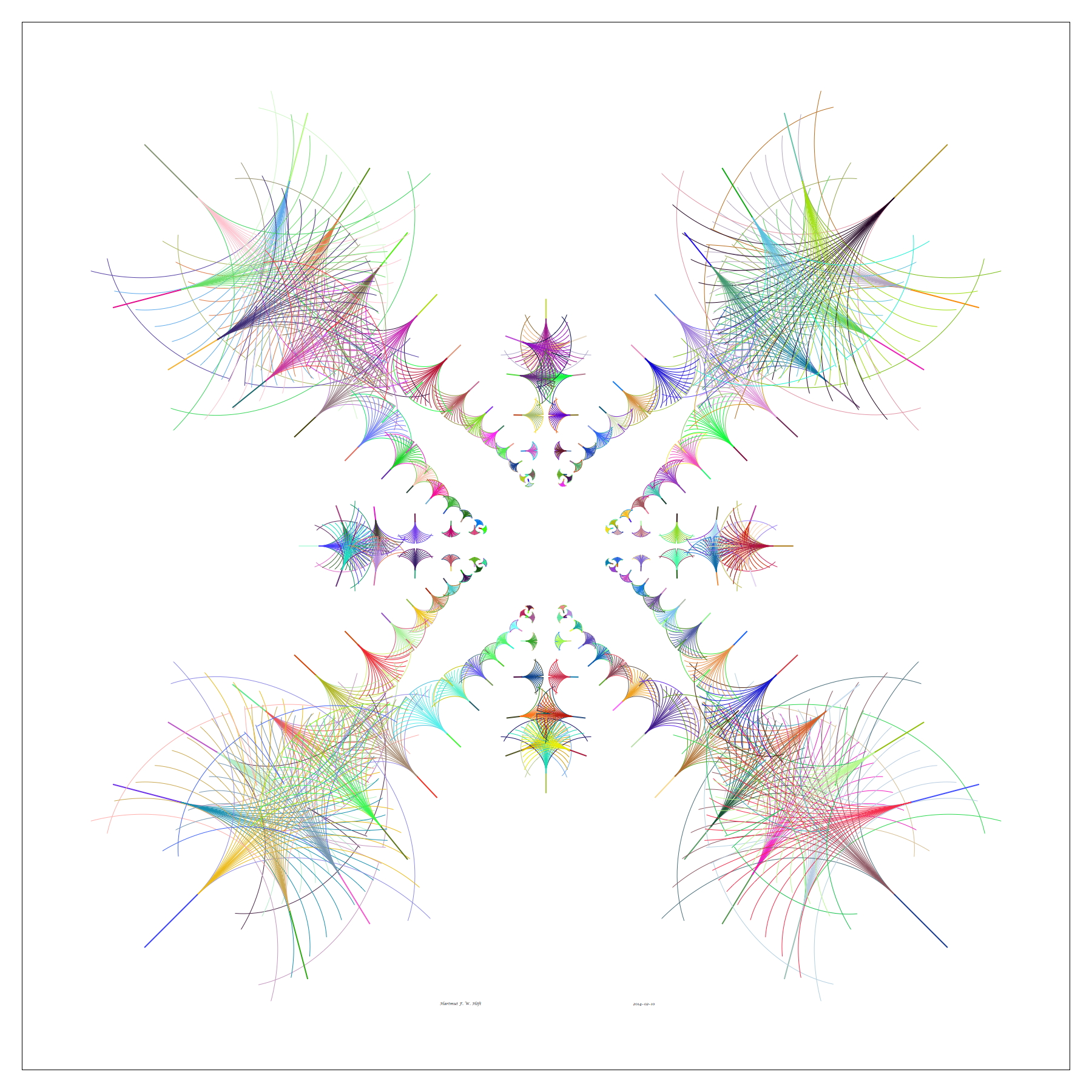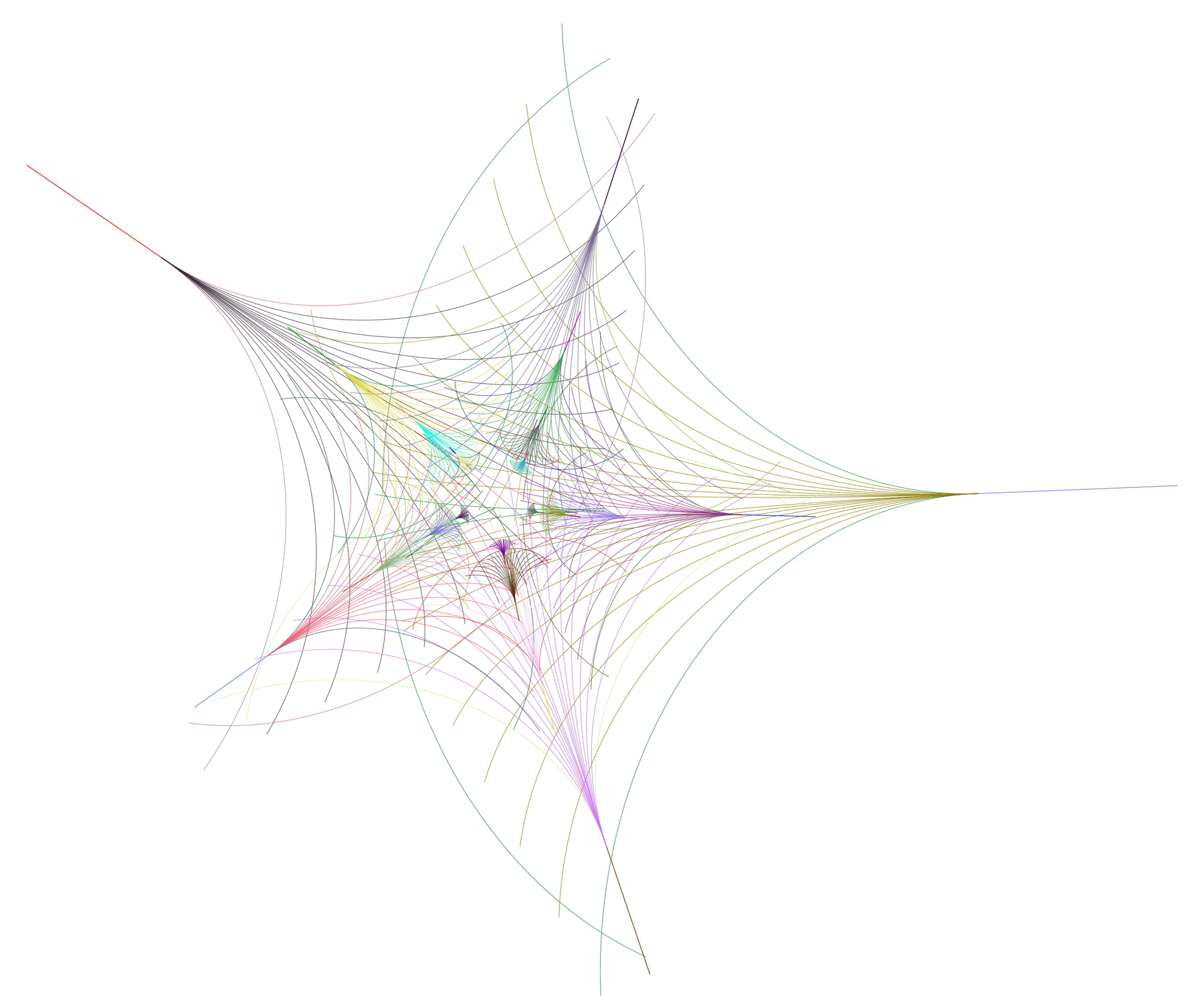# Hartmut F. W. Höft

Professor of Computer Science, Emeritus
Eastern Michigan University
Ypsilanti, Michigan, USA

Many shapes in nature are variations on a single general principle, whether in the animal kingdom, plants or the skies. One such basic 2-dimensional geometric form is the elliptic arc: think of bugs, leaves, pebbles or galaxies. Though there are an infinite variety of elliptic arcs they all are constructed using the same geometric principle. One plant and its shape that has endured for millions of years is the ginkgo leaf. I have always been intrigued by it, and here use elliptic arcs to create an abstract rendering of the ginkgo leaf. In my designs I use scaling, rotation and translation on ginkgo leaves to compute their individual locations and their positions relative to their neighbors. All images are created in Mathematica.Phantom Spiky Diatom
24" x 24" (unframed)
digital print on archival paper
2014

The image contains 145 ginkgo leaves that are placed along a closed curve determined by a sum of the trigonometric functions (sin(2 t))^2 and (cos(4 t))^4. Since one of the parameters is a multiple of the other we see twofold and fourfold symmetries in the image. The curve moves between three concentric circles with radii 2, 5/8, and 4 that are determined by the extremal points on the curve at the respective angles 0, pi/12, and pi/4 in the first quadrant, for example. Also, since the symmetry axis of each ginkgo leaf is along the normal to the curve, you can actually "see" the curve, though it is not drawn. Many actual diatoms have smooth, ellipsoid skeletons; however, spiky diatoms of high rotational symmetry exist in nature.5-Pointed Ginkgo Star
20" x 24" (unframed)
digital print on archival paper
2013

The image contains 21 ginkgo leaves that are placed on the logarithmic spiral r = e^(-t/25); however, the image appears to be a 5-pointed star-like design. The reason for this illusion is twofold: (1) I set the rotational interval between successive ginkgo leaves at 2.5 radians or about 143.24 degrees and (2) the fact that 4xpi - 5x2.5 equals about 0.06637 radians or about 3.8 degrees; in other words: going around five time placing ginkgo leaves gets you almost back to where you started (even after four complete cycles the total angular turn is only about 15 degrees). When you search for the sequence of ginkgo leaves of logarithmically decreasing size (scanning counter-clockwise) you can see the hidden logarithmic spiral.Vanishing Ginkgo Leaves
28" x 16" (unframed)
digital print on archival paper
2014

The image contains 65 ginkgo leaves that are placed along a closed curve determined by a sum of the two trigonometric functions (sint(3 t))^4 and (cos(4 t))^3. Therefore, we can see a (dominating) two-fold symmetry as well as three-fold and four-fold symmetries in the image. Because the curve crosses through the origin eight times, and since size of a ginkgo leaf is proportional to distance from the origin, the ginkgo leaves appear to vanish into the center of the image. The symmetry of the ginkgo leaves in the image is created by an sequence of equidistant angular parameter values that are multiples of a fixed rational fraction of pi. However, since I assign colors randomly to each leaf there is no color symmetry.